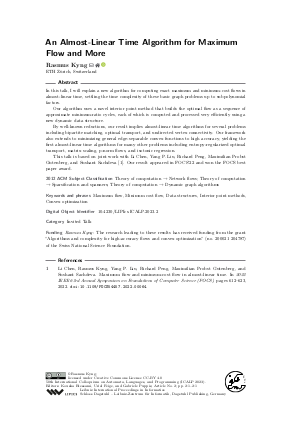Document# An Almost-Linear Time Algorithm for Maximum Flow and More (Invited Talk)

### Author Rasmus Kyng## File

LIPIcs.ICALP.2023.2.pdf
• Filesize: 377 kB
• 1 pages

## Cite As

Rasmus Kyng. An Almost-Linear Time Algorithm for Maximum Flow and More (Invited Talk). In 50th International Colloquium on Automata, Languages, and Programming (ICALP 2023). Leibniz International Proceedings in Informatics (LIPIcs), Volume 261, p. 2:1, Schloss Dagstuhl - Leibniz-Zentrum für Informatik (2023)
https://doi.org/10.4230/LIPIcs.ICALP.2023.2

## Abstract

In this talk, I will explain a new algorithm for computing exact maximum and minimum-cost flows in almost-linear time, settling the time complexity of these basic graph problems up to subpolynomial factors. Our algorithm uses a novel interior point method that builds the optimal flow as a sequence of approximate minimum-ratio cycles, each of which is computed and processed very efficiently using a new dynamic data structure. By well-known reductions, our result implies almost-linear time algorithms for several problems including bipartite matching, optimal transport, and undirected vertex connectivity. Our framework also extends to minimizing general edge-separable convex functions to high accuracy, yielding the first almost-linear time algorithms for many other problems including entropy-regularized optimal transport, matrix scaling, p-norm flows, and isotonic regression. This talk is based on joint work with Li Chen, Yang P. Liu, Richard Peng, Maximilian Probst Gutenberg, and Sushant Sachdeva [Chen et al., 2022]. Our result appeared in FOCS'22 and won the FOCS best paper award.

## Subject Classification

##### ACM Subject Classification
• Theory of computation → Network flows
• Theory of computation → Sparsification and spanners
• Theory of computation → Dynamic graph algorithms
##### Keywords
• Maximum flow
• Minimum cost flow
• Data structures
• Interior point methods
• Convex optimization

## Metrics

• Access Statistics
• Total Accesses (updated on a weekly basis)
0

## References

1. Li Chen, Rasmus Kyng, Yang P. Liu, Richard Peng, Maximilian Probst Gutenberg, and Sushant Sachdeva. Maximum flow and minimum-cost flow in almost-linear time. In 2022 IEEE 63rd Annual Symposium on Foundations of Computer Science (FOCS), pages 612-623, 2022. URL: https://doi.org/10.1109/FOCS54457.2022.00064.
X

Feedback for Dagstuhl Publishing